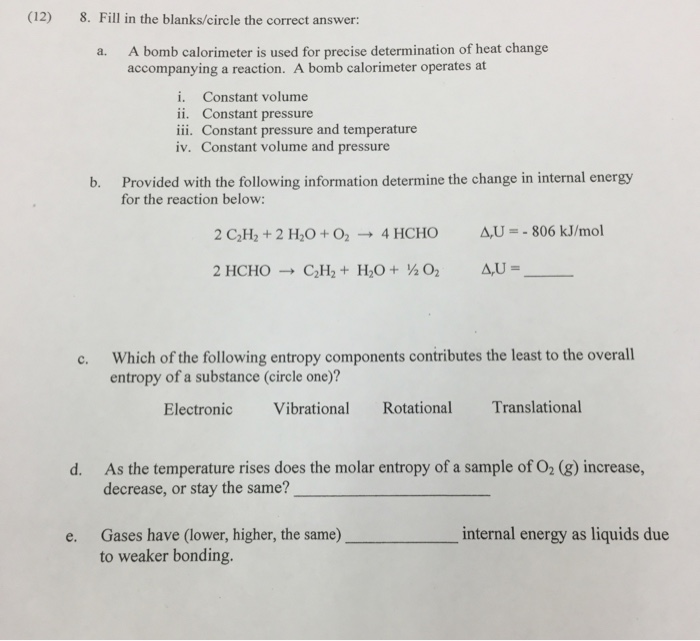# Question & Answer: a. A bomb calorimeter is used for precise determination of heat change accompanying a…..a. A bomb calorimeter is used for precise determination of heat change accompanying a reaction. A bomb calorimeter operates at i. Constant volume ii. Constant pressure iii. Constant pressure and temperature iv. Constant volume and pressure b. Provided with the following information determine the change in internal energy for the reaction below: 2 C_2 H_2 + 2 H_2 O + O_2 rightarrow 4 HCHO delta_r U = -806 kJ/mol @ HCHO rightarrow C_2 H-2 + H_2 O + 1/2 O_2 delta_r U = _______ c. Which of the following entropy components contributes the least to the overall entropy of a substance?. Electronic Vibrational Rotational Translational d. As the temperature rises does the molar entropy of a sample of O_2 (g) increase, decrease, or stay the same? _______ e. Gases have (lower, higher, the same) _________ internal energy as liquids due to weaker bonding.

a. A bomb calorimeter is a type of constant volume calorimeter used to measure the heat of combustion of a reaction.

Don't use plagiarized sources. Get Your Custom Essay on
Question & Answer: a. A bomb calorimeter is used for precise determination of heat change accompanying a…..
GET AN ESSAY WRITTEN FOR YOU FROM AS LOW AS \$13/PAGE

i. constant volume

b. Divide the first equation by 2 to get the second equation. So, the ΔrU = (-806 kJ/mol) / 2 = -403 kJ/mol.

The reactants and products in first equation are exchanged in second equation and the sign of change in internal energy has to be changed. So, the ΔrU = 403 kJ/mol.

The change in internal energy for the reaction is ΔrU = 403 kJ/mol.

c. Entropy is due to the motional energy of molecules. Vibrational, Rotational and Translational are motion types.

So, the entropy component contributing least to the overall entropy of the substance is electronic and the answer is

Electronic

d. As the temperature is raised, the entropy of the system increases. This is because raising the temperature increases the energy. As a result, the molecules are excited more and the randomness or entropy increases. So, the answer is increase.

e. Gases have higher internal energy as liquids due to weaker bonding.

This is because in gases the molecules are loosely packed, the molecules can move around faster and have a higher internal energy compared to liquids where the molecules are tightly packed compared to gases.